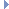﻿ MDynSweptLine Class Reference

# MDynSweptLine Class Reference

MDynSweptLine Class Reference

`#include <MDynSweptLine.h>`

## Class Description

Class for evaluating curve segments as lines over time.

A MDynSweptLine provides access to a curve segment defined as a line. It can only be accessed with the MFnDynSweptGeometryData class that is provided as an output from the geoConnector node.

The class provides parametric time evaluation for a curve. Time is in the range 0 to 1, where 1 represents the current frame and 0 represents the previous frame. In this way you can get interpolated values of a curve in motion.Examples:

## Public Member Functions

MDynSweptLine ()
The class constructor.

~MDynSweptLine ()
The class destructor.

MVector vertex (int vertexId, double t=1.0)
Return the vertex requested by id, at the parametric time value. More...

MVector normal (double x, double y, double z, double t=1.0)
Given a parametric time specified by 't' and a vector, returns a normalized vector perpendicular to the tangent, and rotated into the plane defined by the tangent and vector argument. More...

MVector tangent (double t=1.0)
Given a parametric time specified by 't', returns normalized tangent of the line. More...

double length (double t=1.0)
Given a parametric time specified by 't', returns the total length of the line. More...

## Static Public Member Functions

static const char * className ()
Returns the name of this class. More...

## Member Function Documentation

 MVector vertex ( int vertexId, double t = `1.0` )

Return the vertex requested by id, at the parametric time value.

Parameters
 [in] vertexId index 0 or 1 [in] t time value in the range 1.0 to 0.0 t=1.0 is the current frame t=0.0 is the previous frame
Returns
The vertex positionExamples:
 MVector normal ( double x, double y, double z, double t = `1.0` )

Given a parametric time specified by 't' and a vector, returns a normalized vector perpendicular to the tangent, and rotated into the plane defined by the tangent and vector argument.

Parameters
 [in] x x component of a vector [in] y y component of a vector [in] z z component of a vector [in] t time value in the range 1.0 to 0.0 t=1.0 is the current frame t=0.0 is the previous frame
Returns
A normalized vector
 MVector tangent ( double t = `1.0` )

Given a parametric time specified by 't', returns normalized tangent of the line.

Parameters
 [in] t time value in the range 1.0 to 0.0 t=1.0 is the current frame t=0.0 is the previous frame
Returns
A normalized vector
 double length ( double t = `1.0` )

Given a parametric time specified by 't', returns the total length of the line.

Parameters
 [in] t time value in the range 1.0 to 0.0 t=1.0 is the current frame t=0.0 is the previous frame
Returns
Total line length
 const char * className ( )
static

Returns the name of this class.

Returns
The name of this class.

The documentation for this class was generated from the following files:
• MDynSweptLine.h
• MDynSweptLine.cpp